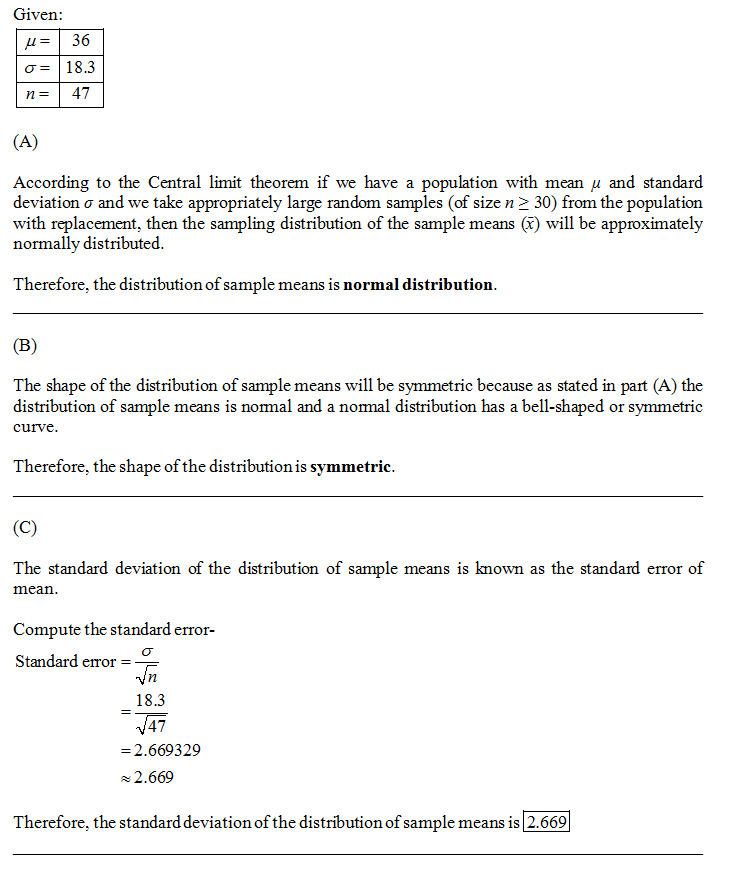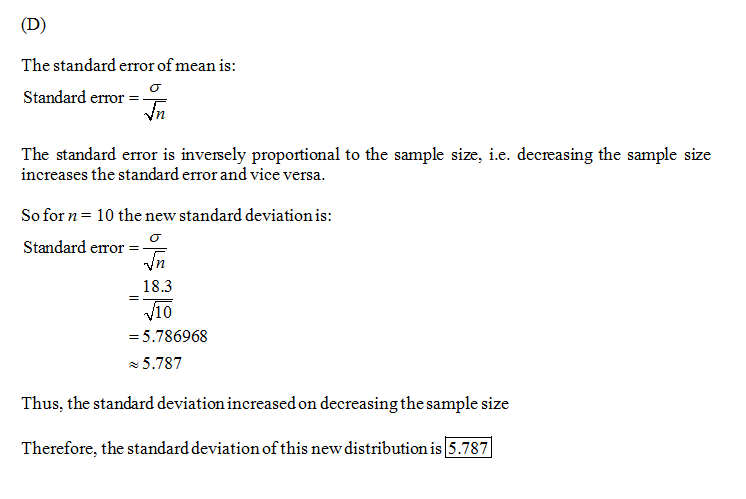In: Math

# The distribution of the number of eggs laid by a certain species of hen during their...

The distribution of the number of eggs laid by a certain species of hen during their breeding period has a mean of 36 eggs with a standard deviation of 18.3. Suppose a group of researchers randomly samples 47 hens of this species, counts the number of eggs laid during their breeding period, and records the sample mean. They repeat this 1,000 times, and build a distribution of sample means. A) What is this distribution called? B) Would you expect the shape of this distribution to be symmetric, right skewed, or left skewed? Explain your reasoning. Left skewed, because the population distribution is left skewed. Left skewed, because according to the central limit theorem this distribution is approximately normal. Left skewed, because the population standard deviation is smaller than the population mean. Symmetric, because the population distribution is symmetric. Symmetric, because according to the central limit theorem this distribution is approximately normal. Symmetric, because the population standard deviation is smaller than the population mean. Right skewed, because the population distribution is right skewed. Right skewed, because according to the central limit theorem this distribution is approximately normal. Right skewed, because the population standard deviation is smaller than the population mean. C) Calculate the standard deviation of this distribution (i.e. the standard error). D) Suppose the researchers' budget is reduced and they are only able to collect random samples of 10 hens. The sample mean of the number of eggs is recorded, and we repeat this 1,000 times, and build a new distribution of sample means. What would be the standard error of this new distribution?

## Solutions

##### Expert Solution## Related Solutions

##### A hen lays N eggs, where N has the Poisson distribution with parameter λ. Each egg...
A hen lays N eggs, where N has the Poisson distribution with parameter λ. Each egg hatches with probability p independently of the other eggs. Let K be the number of chicks. What is the covariance between N and K?
##### The owner of Britten's Egg Farm wants to estimate the mean number of eggs laid per...
The owner of Britten's Egg Farm wants to estimate the mean number of eggs laid per chicken. A sample of 16 chickens shows they laid an average of 18 eggs per month with a standard deviation of 5 eggs per month.   a-2. What is the best estimate of this value? ANS ________ For a 90% confidence interval, what is the value of t? (Round the final answer to 3 decimal places.) The value of t is______    d. Develop the...
##### The owner of Britten's Egg Farm wants to estimate the mean number of eggs laid per...
The owner of Britten's Egg Farm wants to estimate the mean number of eggs laid per chicken. A sample of 30 chickens shows they laid an average of 25 eggs per month with a standard deviation of 12 eggs per month.   a-2. What is the best estimate of this value? c. For a 80% confidence interval, what is the value of t? (Round the final answer to 3 decimal places.) The value of t is  .    d. Develop the 80%...
##### The owner of Britten's Egg Farm wants to estimate the mean number of eggs laid per...
The owner of Britten's Egg Farm wants to estimate the mean number of eggs laid per chicken. A sample of 34 chickens shows they laid an average of 27 eggs per month with a standard deviation of 14 eggs per month.   a-1. What is the value of the population mean? (Click to select)  It is unknown.  27.00  14 a-2. What is the best estimate of this value? b-1. Explain why we need to use the t distribution. (Click to select)  Use the t distribution...
##### The owner of Britten's Egg Farm wants to estimate the mean number of eggs laid per...
The owner of Britten's Egg Farm wants to estimate the mean number of eggs laid per chicken. A sample of 20 chickens shows they laid an average of 21 eggs per month with a sample standard deviation of 2.99 eggs per month. (a-1) What is the value of the population mean?    (Click to select)21, 2.99, or It is unknown.    (a-2) What is the best estimate of this value?    (c) For a 99 percent confidence interval, the value...
##### The owner of Britten's Egg Farm wants to estimate the mean number of eggs laid per...
The owner of Britten's Egg Farm wants to estimate the mean number of eggs laid per chicken. A sample of 20 chickens shows they laid an average of 20 eggs per month with a standard deviation of 2 eggs per month. Develop the 95% confidence interval for the population mean. Would it be reasonable to conclude that the population mean is 21 eggs? What about 25 eggs?
##### The owner of Britten's Egg Farm wants to estimate the mean number of eggs laid per...
The owner of Britten's Egg Farm wants to estimate the mean number of eggs laid per chicken. A sample of 20 chickens shows they laid an average of 19 eggs per month with a sample standard deviation of 2.91 eggs per month. (a-1) What is the value of the population mean?    (Click to select)2.9119It is unknown.    (a-2) What is the best estimate of this value?    (c) For a 95 percent confidence interval, the value of t is...
##### A poultry farmer wants to know which breed of hen lays more eggs. A random sample...
A poultry farmer wants to know which breed of hen lays more eggs. A random sample of 60 values of the average daily egg production by the Rhode Island Red hens had a sample mean of 0.765 egg per hen and a sample standard deviation of 0.11. A random sample of 50 values of the average daily egg production by the Plymouth Rock hens had a mean of 0.559 egg per hen and a standard deviation of 0.09. Determine the...
##### In a certain species of moth, there are two variations in the number of black spots...
In a certain species of moth, there are two variations in the number of black spots on the wings, either 2 or 4, which appear to be the result of a single gene. Scientists hypothesize that 4 spots is dominant to two spots. They cross two individuals that appear to be heterozygous for this trait and 140 offspring are produced. If 4 spots is in fact dominant: 1. how many would be expected to display 4 spots? 2.  how many would...
##### A certain retail store bases its staffing on the number of customers that arrive during certain...
A certain retail store bases its staffing on the number of customers that arrive during certain time slots. Based on prior experience this store expects 32% of its customers between 8:00 am and 12:00 pm; 21% of its customers between 12:00 pm and 4:00 pm; 35% of its customers between 4:00 pm and 8:00 pm; and 12% of its customers between 8:00 pm and midnight. On a certain day, the store had 214, 198, 276, and 134 customers in those...